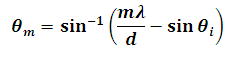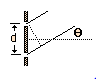# Diffraction Grating Calculator

This Calctown Calculator calculates the the angle for a double slit diffraction grating.

nm
nm
deg

#### Result

degClick here to view image

where

n = integer (order of maxima or peak)

λ = wavelength of X ray

d = slit separation

θi = incident angle

θm = maxima angle